# Mathematics - Signals And Systems - Exercises on Continuous-Time Fourier Series

in StemSocial2 months ago[Image1]

## Introduction

Hey it's a me again @drifter1!

Today we continue with my mathematics series about Signals and Systems in order to cover Exercises on Continuous-Time Fourier Series.

So, without further ado, let's dive straight into it!

## Continuous-Time Fourier Series Recap

Let's quickly refresh our knowledge on the Fourier Series. The Fourier Series is a way of representing periodic signals as a sum of weighted, harmonically related, complex exponentials. This procedure can be split into two parts:

• Fourier Synthesis
• Fourier Analysis

The Fourier Synthesis equation, which is the sum that forms the signal, is: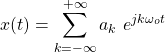whilst the Fourier Analysis equation, which gives us the corresponding coefficients, is: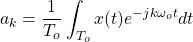## Fourier Series Coefficients Calculation [Based on 7.3 from Ref1]

For a fundamental frequency ωo = 2π, find the Fourier series coefficients for each of the following signals: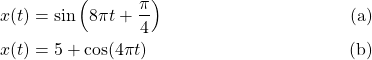### Solution

#### (a)

From Euler's Formula we know that a complex exponential can be turned into a sum of a sine and cosine trigonometric function, as follows: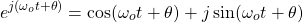As such, the cosine represents the real part of the complex exponential, whilst the sine represents the imaginary part. Due to conjugate symmetry, relating the sine to the complex exponential is as simple as: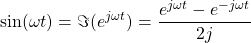Thus, the signal x(t) can be re-written as: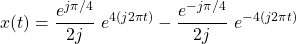Because the fundamental frequency is chosen to be , the Fourier Series is of the form: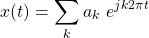From the Euler expansion that we did for the signal, its easy to notice that only k = 4 and k = - 4 give non-zero results. So, the final coefficients are: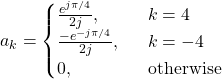#### (b)

The cosine signal can be represented in a similar sense using the following formula: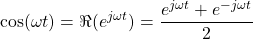Using this representation the signal x(t) can be defined as: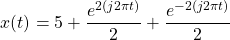which leads us to the following coefficients: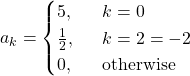## Fourier Series from Signal Graph [Based on 7.4 from Ref1]

Consider the following periodic signal: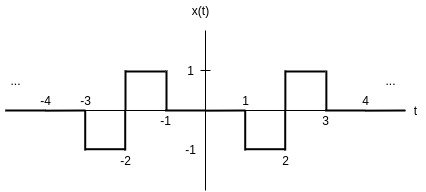Let's evaluate the Fourier series analysis equation.

### Solution

From the graph its easy to notice that the period of the signal is To = 4 and so the fundamental frequency is: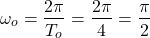Chosing a period of integration from - 2 to 2, can give us the Fourier coefficients as follows: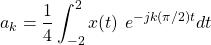But, x(t) is inverse symmetrical when taken at - 2 to -1 and 1 to 2 correspondingly. Let's also note that x(0) = 0. Thus, its possible to re-write the formula as: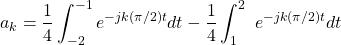where x(t) is completely gone.

Let's continue with the calculations to find the final equation for the coefficients...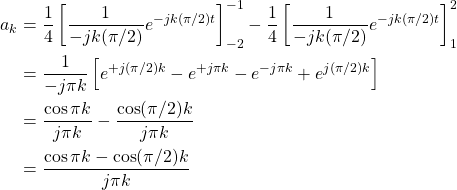In order to calculate the value of ao, because it leads to 0 / 0, L'Hopital's rule can be applied.

## RESOURCES:

### Images

Mathematical equations used in this article were made using quicklatex.

Block diagrams and other visualizations were made using draw.io

## Final words | Next up

And this is actually it for today's post!

Next time we will get into exercises on the continuous-time Fourier transform!

See Ya!Keep on drifting!